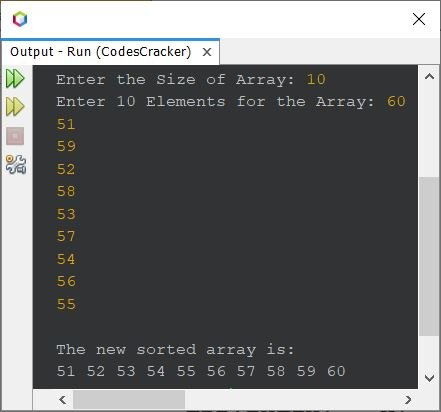# Java Program for Selection Sort

This article covers a program in Java to perform selection sort. The selection sort code in Java is created for both, ascending and descending.

Note - In selection sort, first the smallest element gets selected and moved to the very first index, then second smallest element gets selected and moved to second index, and so on.

If you're not aware about, how the selection sort works ?
then refer to Selection Sort Algorithm and Example. Now let's create the program.

## Java Code for Selection Sort in Ascending Order

The question is, write a Java program for selection sort in ascending order. The array on which the selection sort gets performed, must be received by user at run-time of the program. The program given below is its answer:

```import java.util.Scanner;

public class CodesCracker
{
public static void main(String[] args)
{
int tot, i, j, count, small, index=0, x;
Scanner scan = new Scanner(System.in);

System.out.print("Enter the Size of Array: ");
tot = scan.nextInt();
int[] arr = new int[tot];

System.out.print("Enter " +tot+ " Elements for the Array: ");
for(i=0; i<tot; i++)
arr[i] = scan.nextInt();

for(i=0; i<(tot-1); i++)
{
count=0;
small = arr[i];
for(j=(i+1); j<tot; j++)
{
if(small>arr[j])
{
small = arr[j];
count++;
index = j;
}
}
if(count!=0)
{
x = arr[i];
arr[i] = small;
arr[index] = x;
}
}

System.out.println("\nThe new sorted array is: ");
for(i=0; i<tot; i++)
System.out.print(arr[i]+ " ");

}
}```

The snapshot given below shows the sample run of above program with user input 10 as size of array, and 60, 51, 59, 52, 58, 53, 57, 54, 56, 55 as its ten elements, to sort the array in ascending order, using selection sort technique:## Java Code for Selection Sort in Descending Order

To perform selection sort in Java, but in descending order, you need to change only one character from above program. That is:
Replace the following code, from above program:

`if(small>arr[j])`

with the code given below:

`if(small<arr[j])`

To void misunderstanding, change the name of the variable small with big. Rest of all the codes, remains same as of previous program.

Can you believe, just a matter of a single character, the whole program gets reversed :).

#### Same Program in Other Languages

Java Online Test

« Previous Program Next Program »